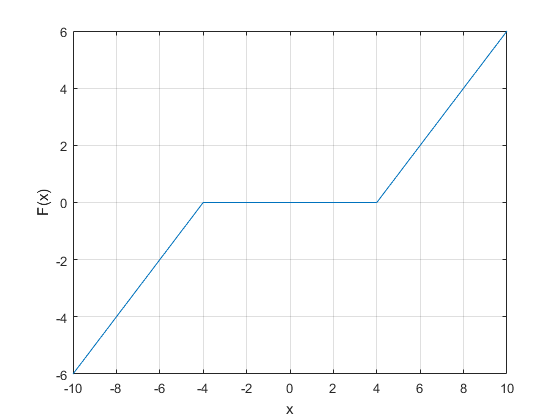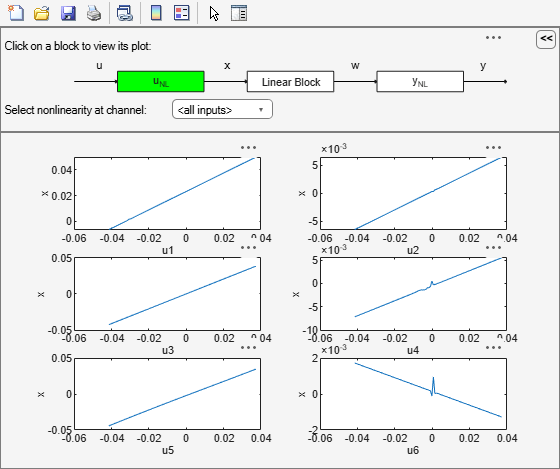Create a dead-zone nonlinearity estimator object

## Description

`idDeadZone` is an object that stores the dead-zone nonlinearity estimator for estimating Hammerstein-Wiener models.

Use `idDeadZone` to define a nonlinear function $y=F\left(x,\theta \right)$, where y and x are scalars, and θ represents the parameters a and b, which define the zero interval.

The dead-zone nonlinearity function has the following characteristics:

For example, in the following plot, the dead-zone is in the interval `[-4,4]`.The value `F(x)` is computed by `evaluate(NL,x)`, where `NL` is the `idDeadZone` object.

For `idDeadZone` object properties, see Properties.

## Creation

### Syntax

``NL = idDeadZone``
``NL = idDeadZone('ZeroInterval',[a,b])``

### Description

example

````NL = idDeadZone` creates a default dead-zone nonlinearity estimator object for estimating Hammerstein-Wiener models. The interval in which the dead-zone exists (zero interval) is set to `[NaN NaN]`. The initial value of the zero interval is determined from the estimation data range, during estimation using `nlhw`. Use dot notation to customize the object properties, if needed.```

example

````NL = idDeadZone('ZeroInterval',[a,b])` creates a dead-zone nonlinearity estimator object initialized with zero interval, `[a,b]`.Alternatively, use `NL = idDeadZone([a,b])`.```

## Properties

expand all

Zero interval of the dead-zone, specified as a 2–element row vector of doubles.

The dead-zone nonlinearity is initialized at the interval `[a,b]`. The interval values are adjusted to the estimation data by `nlhw`. To remove the lower limit, set `a` to `-Inf`. The lower limit is not adjusted during estimation. To remove the upper limit, set `b` to `Inf`. The upper limit is not adjusted during estimation.

When the interval is `[NaN NaN]`, the initial value of the zero interval is determined from the estimation data range during estimation using `nlhw`.

Example: `[-2 1]`

Zero interval of the dead-zone, specified as a 2–element row vector of doubles.

Option to fix or free the parameters of `ZeroInterval`, specified as a 2–element logical row vector. When you set an element of `Free` to `false`, the corresponding value in `ZeroInterval` remains fixed during estimation to the initial value that you specify.

## Examples

collapse all

`NL = idDeadZone;`

Specify the zero interval.

`NL.ZeroInterval = [-4,5];`

```load twotankdata; z = iddata(y,u,0.2,'Name','Two tank system'); z1 = z(1:1000);```

Create an `idDeadZone` object, and specify the initial guess for the zero-interval.

`OutputNL = idDeadZone('ZeroInterval',[-0.1 0.1]);`

Estimate model with no input nonlinearity.

`m = nlhw(z1,[2 3 0],[],OutputNL);`

`load motorizedcamera;`

Create an `iddata` object.

`z = iddata(y,u,0.02,'Name','Motorized Camera','TimeUnit','s');`

`z` is an `iddata` object with six inputs and two outputs.

Specify the model orders and delays.

`Orders = [ones(2,6),ones(2,6),ones(2,6)];`

Specify the same nonlinearity estimator for each input channel.

`InputNL = idWaveletNetwork;`

Specify different nonlinearity estimators for each output channel.

` OutputNL = [idDeadZone,idWaveletNetwork];`

Estimate the Hammerstein-Wiener model.

`sys = nlhw(z,Orders,InputNL,OutputNL);`

To see the shape of the estimated input and output nonlinearities, plot the nonlinearities.

`plot(sys)`Click on the input and output nonlinearity blocks on the top of the plot to see the nonlinearities.

## Version History

Introduced in R2007a

expand all